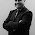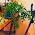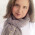MATH 1280 Introduction to Statistics – Assignment 2 – Part 2

Part 2 - Answers for question 5 to 8
Question 5. “Assuming that you read the flowers.csv file into an R object called flower.data, run the following R code (do not paste the ">” character into R) and paste both the command and the output into your answer (you should see five names, each of which should be enclosed in quotes--if you do not see this, try again or contact your instructor):”
> names(flower.data)
Answer:  "Sepal.Length" "Sepal.Width" "Petal.Length" "Petal.Width" "Species"
The code:
> flower.data <- read.csv(“flowers.csv”)
> names(flower.data)

I also change the code, but the result is the same:
> fl.1 <- read.csv("fl1.csv")
> names(fl.1)

Result:
 "Sepal.Length" "Sepal.Width" "Petal.Length" "Petal.Width" "Species"

Question 6. “The number of observations in the "flower.data" data frame is:”
150 observations with 3 different species:
setosa :50
versicolor:50
virginica :50

Illustration for code in statistic

Question 7. “List the variables in the data frame (you can do this by entering the name of the R object that holds that data that you read using the read.csv command--you should have called it flower.data). If you do not see five columns of data, then there was a problem reading the input file--try again or contact your instructor. For each variable identify the type of the variable (factor or numeric).”
The name and type of the 1st variable: "Sepal.Length"….> Numeric
The name and type of the 2nd variable: "Sepal.Width" …..> Numeric
The name and type of the 3nd variable: "Petal.Length" ……> Numeric
The name and type of the 4nd variable:_ "Petal.Width" …..> Numeric
The name and type of the 5nd variable: “Species”…..> Factor

Question 8. “Round the data for the variable Sepal.Length so that it contains integers, then find the frequency of the value 7 (not the relative frequency):”
The frequency is 24
R codes:
> fl.1 <- read.csv("fl1.csv")
> Sepal.Length <- round(fl.1\$Sepal.Length, 0)
> freq<-table(Sepal.Length)
> freq

Result:
Sepal.Length
4 5 6 7 8
5 47 68 24 6
Thus, the frequency of the value 7 is 24

1.Woow Nice article I am like your post. "Perfect "
Good job TanksFor sharing

Buku Vs Youtube Apa Yang Ada Di Benak Anda Kebanyakan berasumsi No..

1.Thank you to visit my article:
MATH 1280 Introduction to Statistics – Assignment 2 – Part 2
@
Modified illustration of the flower

2.MATH 1280, Introduction to Statistics – Assignment 2 – Part 2
@
Question 6. “The number of observations in the "flower.data" data frame is:”

150 observations with 3 different species:
setosa :50
versicolor:50
virginica :50

2.Interesting post :) have a beautiful day :)

1.Thank you to visit my article:
MATH 1280, Introduction to Statistics – Assignment 2 – Part 2
@
Part 2 - Answers for question 5 to 8

Question 5. “Assuming that you read the flowers.csv file into an R object called flower.data, run the following R code (do not paste the ">” character into R) and paste both the command and the output into your answer (you should see five names, each of which should be enclosed in quotes--if you do not see this, try again or contact your instructor):”
> names(flower.data)

Answer:  "Sepal.Length" "Sepal.Width" "Petal.Length" "Petal.Width" "Species"

The code:
> flower.data <- read.csv(“flowers.csv”)
> names(flower.data)

I also change the code, but the result is the same:

> fl.1 <- read.csv("fl1.csv")

> names(fl.1)

Result:

 "Sepal.Length" "Sepal.Width" "Petal.Length" "Petal.Width" "Species"

2.MATH 1280, Introduction to Statistics – Assignment 2 – Part 2
@
Illustration for code in statistic

3.1.ok, I removed it already...

Thank you to visit my article:
MATH 1280, Introduction, to Statistics – Assignment 2 – Part 2
@
Question 6. “The number of observations in the "flower.data" data frame is:”

150 observations with 3 different species:

setosa :50

versicolor:50

virginica :50

2.MATH 1280, Introduction to Statistics – Assignment 2 – Part 2
@
Question 7. “List the variables in the data frame (you can do this by entering the name of the R object that holds that data that you read using the read.csv command--you should have called it flower.data). If you do not see five columns of data, then there was a problem reading the input file--try again or contact your instructor. For each variable identify the type of the variable (factor or numeric).”
The name and type of the 1st variable: "Sepal.Length"….> Numeric
The name and type of the 2nd variable: "Sepal.Width" …..> Numeric
The name and type of the 3nd variable: "Petal.Length" ……> Numeric
The name and type of the 4nd variable:_ "Petal.Width" …..> Numeric
The name and type of the 5nd variable: “Species”…..> Fact

4.İnteresting post 😊 thanks for your sharing 😊

1.hank you to visit my article:
MATH 1280, Introduction, to, Statistics – Assignment 2 – Part 2
@
Question 7. “List the variables in the data frame (you can do this by entering the name of the R object that holds that data that you read using the read.csv command--you should have called it flower.data). If you do not see five columns of data, then there was a problem reading the input file--try again or contact your instructor. For each variable identify the type of the variable (factor or numeric).”
The name and type of the 1st variable: "Sepal.Length"….> Numeric
The name and type of the 2nd variable: "Sepal.Width" …..> Numeric

The name and type of the 3nd variable: "Petal.Length" ……> Numeric

The name and type of the 4nd variable:_ "Petal.Width" …..> Numeric

The name and type of the 5nd variable: “Species”…..> Factor

2.MATH 1280, Introduction to Statistics – Assignment 2 – Part 2
@
Question 8. “Round the data for the variable Sepal.Length so that it contains integers, then find the frequency of the value 7 (not the relative frequency):”

The frequency is 24

R codes:

> fl.1 <- read.csv("fl1.csv")

> Sepal.Length <- round(fl.1\$Sepal.Length, 0)

> freq<-table(Sepal.Length)

> freq

Result:

Sepal.Length

4 5 6 7 8

5 47 68 24 6

Thus, the frequency of the value 7 is 24

5.Interesting lesson.

1.hank you to visit my article:
MATH 1280, Introduction, to, Statistics, Assignment 2 – Part 2
@
Question 8. “Round the data for the variable Sepal.Length so that it contains integers, then find the frequency of the value 7 (not the relative frequency):”

The frequency is 24

R codes:

> fl.1 <- read.csv("fl1.csv")

> Sepal.Length <- round(fl.1\$Sepal.Length, 0)

> freq<-table(Sepal.Length)

> freq

Result:

Sepal.Length

4 5 6 7 8

5 47 68 24 6

Thus, the frequency of the value 7 is 24

2.MATH 1280 Introduction to Statistics – Assignment 2 – Part 2

6.very useful information ☺
greetings
Lili

1.Thank you to visit my article:
MATH 1280 Introduction to Statistics – Assignment 2 – Part 2
@
Modified illustration of the flower

2.MATH 1280, Introduction to Statistics – Assignment 2 – Part 2

7.It's amazing how perfectly God designed flowers, isn't it?

1.Thank you to visit my article:
MATH 1280, Introduction to Statistics – Assignment 2 – Part 2
@
Part 2 - Answers for question 5 to 8

Question 5. “Assuming that you read the flowers.csv file into an R object called flower.data, run the following R code (do not paste the ">” character into R) and paste both the command and the output into your answer (you should see five names, each of which should be enclosed in quotes--if you do not see this, try again or contact your instructor):”
> names(flower.data)

Answer:  "Sepal.Length" "Sepal.Width" "Petal.Length" "Petal.Width" "Species"

The code:
> flower.data <- read.csv(“flowers.csv”)
> names(flower.data)

I also change the code, but the result is the same:

> fl.1 <- read.csv("fl1.csv")

> names(fl.1)

Result:

 "Sepal.Length" "Sepal.Width" "Petal.Length" "Petal.Width" "Species"